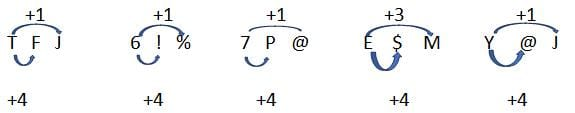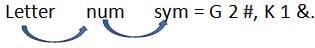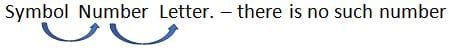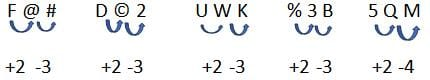CAT  >  Test: Letter Series Type - 5

# Test: Letter Series Type - 5 - CAT

Test Description

## 15 Questions MCQ Test CAT Mock Test Series and 500+ Practice Tests - Test: Letter Series Type - 5

Test: Letter Series Type - 5 for CAT 2023 is part of CAT Mock Test Series and 500+ Practice Tests preparation. The Test: Letter Series Type - 5 questions and answers have been prepared according to the CAT exam syllabus.The Test: Letter Series Type - 5 MCQs are made for CAT 2023 Exam. Find important definitions, questions, notes, meanings, examples, exercises, MCQs and online tests for Test: Letter Series Type - 5 below.
Solutions of Test: Letter Series Type - 5 questions in English are available as part of our CAT Mock Test Series and 500+ Practice Tests for CAT & Test: Letter Series Type - 5 solutions in Hindi for CAT Mock Test Series and 500+ Practice Tests course. Download more important topics, notes, lectures and mock test series for CAT Exam by signing up for free. Attempt Test: Letter Series Type - 5 | 15 questions in 10 minutes | Mock test for CAT preparation | Free important questions MCQ to study CAT Mock Test Series and 500+ Practice Tests for CAT Exam | Download free PDF with solutions
 1 Crore+ students have signed up on EduRev. Have you?
Test: Letter Series Type - 5 - Question 1

### Study the following arrangement and answer the questions below: 5 T J & A F 5 6 % Y J ! 7 @ M # P A 8 + E K A M \$ 4 5 I Which is the 3rd term to the left of the 15th term which is to the left of the 2nd term from the right end?

Detailed Solution for Test: Letter Series Type - 5 - Question 1

5 T J & A | F 5 6 % Y | J ! 7 @ M | # P A 8 + | E K A M \$ | 4 5 I
We divided series in group of each to save time.
Total elements – 28
2nd from right- 27 its 15 left = 27 – 15 = 12 its 3rd left = 12 – 3 = 9
Which is ‘%’.

Test: Letter Series Type - 5 - Question 2

### Study the following arrangement and answer the questions below: 5 T J & A F 5 6 % Y J ! 7 @ M # P A 8 + E K A M \$ 4 5 I How many such consonants are there each of which is followed by a vowel but is not preceded by a letter?

Detailed Solution for Test: Letter Series Type - 5 - Question 2

5 T J & A | F 5 6 % Y | J ! 7 @ M | # P A 8 + | E K A M \$ | 4 5 I
We divided series in group of each to save time.
Consonant followed by vowel but not preceded by letter-
#PA.

Test: Letter Series Type - 5 - Question 3

### Study the following arrangement and answer the questions below: 5 T J & A F 5 6 % Y J ! 7 @ M # P A 8 + E K A M \$ 4 5 I If all the symbols and vowels are dropped from the series, which will be 10th element from the right end?

Detailed Solution for Test: Letter Series Type - 5 - Question 3

5 T J & A | F 5 6 % Y | J ! 7 @ M | # P A 8 + | E K A M \$ | 4 5 I
We divided series in group of each to save time.
Series after given condition – 5 T J F 5 6 Y J 7 M P 8 K M 4 5
10th from right –Y.

Test: Letter Series Type - 5 - Question 4

Study the following arrangement and answer the questions below:
5 T J & A F 5 6 % Y J ! 7 @ M # P A 8 + E K A M \$ 4 5 I

If a vowel gets 1 point, a symbol gets 2 points, all letters that are not vowel get 3 points and the number gets the same number of points that they represent then what is the sum of the series?

Detailed Solution for Test: Letter Series Type - 5 - Question 4

5 T J & A | F 5 6 % Y | J ! 7 @ M | # P A 8 + | E K A M \$ | 4 5 I
We divided series in group of each to save time.
Sum – 86.

Test: Letter Series Type - 5 - Question 5

Study the following arrangement and answer the questions below:
5 T J & A F 5 6 % Y J ! 7 @ M # P A 8 + E K A M \$ 4 5 I

Four of the five sequence are alike in some manner. Find the odd one out?

Detailed Solution for Test: Letter Series Type - 5 - Question 5

5 T J & A | F 5 6 % Y | J ! 7 @ M | # P A 8 + | E K A M \$ | 4 5 I
We divided series in group of each to save time.Test: Letter Series Type - 5 - Question 6

Study the following arrangement and answer the questions below:
2 R 8 C F 8 E \$ G 2 # 4 9 L C % B < K 1 & A W ? P E = Q @ 7 F 6

If the above sequence is written in reverse order, then which element will be 5th to the right of the 15th element from the right end?

Detailed Solution for Test: Letter Series Type - 5 - Question 6

2 R 8 C F 8 E \$ | G 2 # 4 9 | L C % B < | K 1 & A W | ? P E = Q | @ 7 F 6
15th from right – 32 + 1 – 15 = 18th from left. Its 5th right = 18 + 5 = 23rd if series reversed – 1 + 32 -23 = 10th. Which is 2.

Test: Letter Series Type - 5 - Question 7

Study the following arrangement and answer the questions below:
2 R 8 C F 8 E \$ G 2 # 4 9 L C % B < K 1 & A W ? P E = Q @ 7 F 6

How many such numbers are there in the above sequence, each of which is immediately preceded by a constant and immediately followed by a symbol?

Detailed Solution for Test: Letter Series Type - 5 - Question 7

2 R 8 C F 8 E \$ | G 2 # 4 9 | L C % B < | K 1 & A W | ? P E = Q | @ 7 F 6Test: Letter Series Type - 5 - Question 8

Study the following arrangement and answer the questions below:
2 R 8 C F 8 E \$ G 2 # 4 9 L C % B < K 1 & A W ? P E = Q @ 7 F 6

Which element is 7th to the left of 16th element from the left in the above sequence?

Detailed Solution for Test: Letter Series Type - 5 - Question 8

2 R 8 C F 8 E \$ | G 2 # 4 9 | L C % B < | K 1 & A W | ? P E = Q | @ 7 F 6
7th left to 16th from left – 16 – 7 = 9th which is G.

Test: Letter Series Type - 5 - Question 9

Study the following arrangement and answer the questions below:
2 R 8 C F 8 E \$ G 2 # 4 9 L C % B < K 1 & A W ? P E = Q @ 7 F 6

If first half of the series is written in reverse order, then which element will be 21st from the right end?

Detailed Solution for Test: Letter Series Type - 5 - Question 9

2 R 8 C F 8 E \$ | G 2 # 4 9 | L C % B < | K 1 & A W | ? P E = Q | @ 7 F 6
21st from right means – second half + 5th from right in first half, that means 5th from left in original series which if – F

Test: Letter Series Type - 5 - Question 10

Study the following arrangement and answer the questions below:
2 R 8 C F 8 E \$ G 2 # 4 9 L C % B < K 1 & A W ? P E = Q @ 7 F 6

If the second half of the series is written in reverse order, then which element will be 21st from the right end?

Detailed Solution for Test: Letter Series Type - 5 - Question 10

2 R 8 C F 8 E \$ | G 2 # 4 9 | L C % B < | K 1 & A W | ? P E = Q | @ 7 F 6
21st from right means- second half +5th from right in first half,
That means 12th from left which is 4.

Test: Letter Series Type - 5 - Question 11

Study the following arrangement and answer the questions below:
E 4 B % R 3 A 6 # F H @ I 2 D 9 © K U \$ W 1 M P 5 * Q 8 T

If all the numbers are dropped from the above arrangement, Which one of the following will be the 9th to the left of W?

Detailed Solution for Test: Letter Series Type - 5 - Question 11

E 4 B % R 3 A 6 | # F H @ I | 2 D 9 © K | U \$ W 1 M | P 5 * Q 8 T |
W = 21st , its 9th left = 21 - 9 = 12, numbers in between = 2,
Therefore element is F.

Test: Letter Series Type - 5 - Question 12

Study the following arrangement and answer the questions below:
E 4 B % R 3 A 6 # F H @ I 2 D 9 © K U \$ W 1 M P 5 * Q 8 T

How many such numbers are there in the above arrangement each of which is immediately preceded by a symbol and immediately followed by a letter?

Detailed Solution for Test: Letter Series Type - 5 - Question 12

E 4 B % R 3 A 6 | # F H @ I | 2 D 9 © K | U \$ W 1 M | P 5 * Q 8 T |Test: Letter Series Type - 5 - Question 13

Study the following arrangement and answer the questions below:
E 4 B % R 3 A 6 # F H @ I 2 D 9 © K U \$ W 1 M P 5 * Q 8 T

Which of the following is 5th to the right of 18th from the right?

Detailed Solution for Test: Letter Series Type - 5 - Question 13

E 4 B % R 3 A 6 | # F H @ I | 2 D 9 © K | U \$ W 1 M | P 5 * Q 8 T |
18th from right = 29 + 1 – 18 = 12th from left, its 5th right = 12 + 5 = 17th = ©.

Test: Letter Series Type - 5 - Question 14

Study the following arrangement and answer the questions below:
E 4 B % R 3 A 6 # F H @ I 2 D 9 © K U \$ W 1 M P 5 * Q 8 T

Which of the following is 4th to the right of 12th from the left end?

Detailed Solution for Test: Letter Series Type - 5 - Question 14

4 B % R 3 A 6 | # F H @ I | 2 D 9 © K | U \$ W 1 M | P 5 * Q 8 T |
4th right to 12th from left = 12 + 4 = 16th = 9.

Test: Letter Series Type - 5 - Question 15

Study the following arrangement and answer the questions below:
E 4 B % R 3 A 6 # F H @ I 2 D 9 © K U \$ W 1 M P 5 * Q 8 T

Four of the below five are alike in a certain way. Find the odd one?

Detailed Solution for Test: Letter Series Type - 5 - Question 15

4 B % R 3 A 6 | # F H @ I | 2 D 9 © K | U \$ W 1 M | P 5 * Q 8 T |## CAT Mock Test Series and 500+ Practice Tests

2 videos|73 docs|469 tests
Information about Test: Letter Series Type - 5 Page
In this test you can find the Exam questions for Test: Letter Series Type - 5 solved & explained in the simplest way possible. Besides giving Questions and answers for Test: Letter Series Type - 5, EduRev gives you an ample number of Online tests for practice

## CAT Mock Test Series and 500+ Practice Tests

2 videos|73 docs|469 tests

### How to Prepare for CAT

Read our guide to prepare for CAT which is created by Toppers & the best Teachers